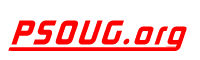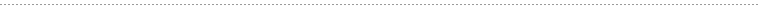Quick Search:JavaScript: A JavaScript implementation of the famous sprintf() function Jump to: Select a Location PSOUG Home Page Oracle Code Library mySQL Code Library PHP Code Library JavaScript Code Library Oracle Terms & Definitions Oracle Error Codes Advanced Code Search Contact UsSnippet Name: A JavaScript implementation of the famous sprintf() function

Description: This is a lightweight yet powerful JavaScript implementation of the famous sprintf() function. It is based on it's PHP counterpart.

It's prototype is simple:

string sprintf(string format , [mixed arg1 [, mixed arg2 [ ,...]]]);

The placeholders in the format string are marked by "%" and are followed by one or more of these elements, in this order:

* An optional "+" sign that forces to preceed the result with a plus or minus sign on numeric values. By default, only the "-" sign is used on negative numbers.
* An optional padding specifier that says what character to use for padding (if specified). Possible values are 0 or any other character precedeed by a '. The default is to pad with spaces.
* An optional "-" sign, that causes sprintf to left-align the result of this placeholder. The default is to right-align the result.
* An optional number, that says how many characters the result should have. If the value to be returned is shorter than this number, the result will be padded.
* An optional precision modifier, consisting of a "." (dot) followed by a number, that says how many digits should be displayed for floating point numbers. When used on a string, it causes the result to be truncated.
* A type specifier that can be any of:

• % - print a literal "%" character
• b - print an integer as a binary number
• c - print an integer as the character with that ASCII value
• d - print an integer as a signed decimal number
• e - print a float as scientific notation
• u - print an integer as an unsigned decimal number
• f - print a float as is
• o - print an integer as an octal number
• s - print a string as is
• x - print an integer as a hexadecimal number (lower-case)
• X - print an integer as a hexadecimal number (upper-case)

Argument swapping

You can also swap the arguments. That is, the order of the placeholders doesn't have to match the order of the arguments. You can do that by simply indicating in the format string which arguments the placeholders refer to:

sprintf("The first 4 letters of the english alphabet are: %4\$s, %3\$s, %1\$s and %2\$s", "c", "d", "b", "a");

And, of course, you can repeat the placeholders without having to increase the number of arguments.

Comment: (none)

Language: JAVASCRIPT
Highlight Mode: JAVASCRIPT

```// Implementation of "sprintf()" for JavaScript 0.6
// Copyright (c) Alexandru Marasteanu <alexaholic [at) gmail (dot] com>

FUNCTION str_repeat(i, m) {
FOR (VAR o = []; m > 0; o[--m] = i);
RETURN o.join('');
}

FUNCTION sprintf() {
VAR i = 0, a, f = arguments[i++], o = [], m, p, c, x, s = '';
WHILE (f) {
IF (m = /^[^\x25]+/.exec(f)) {
o.push(m);
}
ELSE IF (m = /^\x25{2}/.exec(f)) {
o.push('%');
}
ELSE IF (m = /^\x25(?:(\d+)\\$)?(\+)?(0|'[^\$])?(-)?(\d+)?(?:\.(\d+))?([b-fosuxX])/.exec(f)) {
IF (((a = arguments[m || i++]) == NULL) || (a == undefined)) {
THROW('Too few arguments.');
}
IF (/[^s]/.test(m) && (TYPEOF(a) != 'number')) {
THROW('Expecting number but found ' + TYPEOF(a));
}
SWITCH (m) {
CASE 'b': a = a.toString(2); BREAK;
CASE 'c': a = String.fromCharCode(a); BREAK;
CASE 'd': a = parseInt(a); BREAK;
CASE 'e': a = m ? a.toExponential(m) : a.toExponential(); BREAK;
CASE 'f': a = m ? parseFloat(a).toFixed(m) : parseFloat(a); BREAK;
CASE 'o': a = a.toString(8); BREAK;
CASE 's': a = ((a = String(a)) && m ? a.substring(0, m) : a); BREAK;
CASE 'u': a = Math.abs(a); BREAK;
CASE 'x': a = a.toString(16); BREAK;
CASE 'X': a = a.toString(16).toUpperCase(); BREAK;
}
a = (/[def]/.test(m) && m && a >= 0 ? '+'+ a : a);
c = m ? m == '0' ? '0' : m.charAt(1) : ' ';
x = m - String(a).length - s.length;
p = m ? str_repeat(c, x) : '';
o.push(s + (m ? a + p : p + a));
}
ELSE {
THROW('Huh ?!');
}
f = f.substring(m.length);
}
RETURN o.join('');
}
```